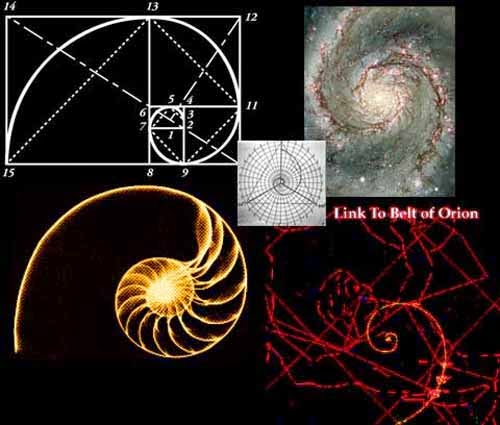## Sunday, June 1, 2014

### Fibonacii

The Fibonacci sequence is possibly the most simple recurrence relation occurring in nature. It is 0,1,1,2,3,5,8,13,21,34,55,89, 144… each number equals the sum of the two numbers before it, and the difference of the two numbers succeeding it. It is an infinite sequence which goes on forever as it develops.
The Golden Ratio/Divine Ratio or Golden Mean
The quotient of any Fibonacci number and it’s predecessor approaches Phi, represented as ϕ (1.618), the Golden ratio. Thequotient of any Fibonacci number and it’s predecessor approaches Phi, represented as ϕ (1.618), the Golden ratio. The Golden Ratio is best understood geometrically by the golden rectangle. A rectangle unevenly divided resulting into one square and one rectangle, the square’s sides would have the ratio of 1:1, and the new rectangle would be exactly proportionate to the original rectangle – 1:1.618.
This iteration can continue both ways, infinitely. If you plot a quarter circle inside each of the squares as they reiterate, the golden spiral is formed. The golden spiral is possibly the most simple mathematic pattern that occurs in nature.